login  home  contents  what's new  discussion  bug reports help  links  subscribe  changes  refresh  edit

)abbrev domain TENSALG TensorAlgebra
++ Author: Kurt Pagani
++ Date Created: Thu Jan 30 23:04:02 CET 2020
++ References:
++   https://en.wikipedia.org/wiki/Tensor_algebra
++ Description:
++  Quote Ref:
++  -- Non-commutative polynomials
++  -- Quotients
++  Because of the generality of the tensor algebra, many other algebras
++  of interest can be constructed by starting with the tensor algebra and
++  then imposing certain relations on the generators, i.e. by constructing
++  certain quotient algebras of T(V). Examples of this are the
++  exterior algebra, the symmetric algebra, Clifford algebras,
++  the Weyl algebra and universal enveloping algebras.
++
TensorAlgebra(M,R,B) : Exports == Implementation where
M:FreeModuleCategory(R, B)
R:Ring
B:OrderedSet
OF     ==> OutputForm
NNI    ==> NonNegativeInteger
FMB    ==> FreeMonoid B
CTOF   ==> CoercibleTo OutputForm
FMCRB  ==> FreeModuleCategory(R,FMB)
XFABR  ==> XFreeAlgebra(B,R)
XDPBR  ==> XDistributedPolynomial(B,R)
TERM   ==> Record(k:FMB,c:R)
Exports == Join(FMCRB, XFABR) with
coerce : B -> %
convert : FMB -> OutputForm
Rep := XDPBR
convert(x:FMB):OutputForm ==
x=1$FMB => empty()$OF
length(x)$FMB = 1 => x::OF length(x)$FMB = 2 => tensor(first(x)::OF,rest(x)::OF)$OF tensor(first(x)::OF, convert(rest x)) coerce(x:%):OutputForm == zero? x => empty()$OF
x=1$% => outputForm(1)$OF
if c=1 then cof:=empty()$OF else cof:=c::OF kof:OF:=cof * convert(leadingSupport(x)) zero? reductum(x) => kof kof + reductum(x)::OF )abbrev domain OOBJ OrderedObjectList ++ Description: ++ This domain implements ordered objects such that the type ++ has OrderedSet. Adapted from OrderedVariableList. OrderedObjectList(T:Comparable, ObjectList : List T): Join(OrderedFinite, ConvertibleTo T) with object : T -> Union(%,"failed") ++ object(s) returns a member of the object set or failed == add ObjectList := removeDuplicates ObjectList Rep := PositiveInteger s1, s2 : % convert(s1) : T == ObjectList.((s1::Rep)::PositiveInteger) coerce(s1) : OutputForm == (convert(s1)@T)::OutputForm index i == i::% lookup j == j :: Rep size () == #ObjectList object(exp : T) == for i in 1.. for exp2 in ObjectList repeat if exp = exp2 then return i::PositiveInteger::% "failed" s1 < s2 == s2 <$Rep s1
s1 = s2 == s1 =$Rep s2 latex(x : %) : String == latex(convert(x)@T) hashUpdate!(hs, s) == update!(hs, SXHASH(s)$Lisp)$HashState -- B:=OrderedObjectList(BOP, map(operator,[a,b,c])) -- index(1)$B
-- enumerate()$B -- size()$B
-- object(operator a)$B spad  Compiling FriCAS source code from file /var/lib/zope2.10/instance/axiom-wiki/var/LatexWiki/3277064617359698758-25px001.spad using old system compiler. TENSALG abbreviates domain TensorAlgebra ------------------------------------------------------------------------ initializing NRLIB TENSALG for TensorAlgebra compiling into NRLIB TENSALG compiling exported convert : FreeMonoid B -> OutputForm Time: 0.01 SEC. compiling exported coerce :$ -> OutputForm
Time: 0.01 SEC.
****** Domain: R already in scope
augmenting R: (OrderedAbelianMonoidSup)
****** Domain: R already in scope
augmenting R: (CommutativeRing)
(time taken in buildFunctor:  10)
;;;     ***       |TensorAlgebra| REDEFINED
;;;     ***       |TensorAlgebra| REDEFINED
Time: 0.01 SEC.
Cumulative Statistics for Constructor TensorAlgebra
Time: 0.03 seconds
--------------non extending category----------------------
.. TensorAlgebra(#1,#2,#3) of cat
(|Join| (|FreeModuleCategory| |#2| (|FreeMonoid| |#3|))
(|XFreeAlgebra| |#3| |#2|)
(CATEGORY |domain| (SIGNATURE |coerce| ($|#3|)) (SIGNATURE |convert| ((|OutputForm|) (|FreeMonoid| |#3|))))) has no (|XPolynomialsCat| |#3| |#2|) finalizing NRLIB TENSALG Processing TensorAlgebra for Browser database: --------constructor--------- --->-->TensorAlgebra((coerce (% B))): Not documented!!!! --->-->TensorAlgebra((convert ((OutputForm) (FreeMonoid B)))): Not documented!!!! ; compiling file "/var/aw/var/LatexWiki/TENSALG.NRLIB/TENSALG.lsp" (written 03 NOV 2020 07:33:59 PM): ; /var/aw/var/LatexWiki/TENSALG.NRLIB/TENSALG.fasl written ; compilation finished in 0:00:00.030 ------------------------------------------------------------------------ TensorAlgebra is now explicitly exposed in frame initial TensorAlgebra will be automatically loaded when needed from /var/aw/var/LatexWiki/TENSALG.NRLIB/TENSALG OOBJ abbreviates domain OrderedObjectList ------------------------------------------------------------------------ initializing NRLIB OOBJ for OrderedObjectList compiling into NRLIB OOBJ ****** Domain: T$ already in scope
Local variable Rep type redefined: (Join (OrderedAbelianSemiGroup) (OrderedMonoid) (CommutativeStar) (ConvertibleTo (InputForm)) (CATEGORY domain (SIGNATURE gcd ( $)) (SIGNATURE qcoerce ($ (Integer))))) to (Join (FreeModuleCategory R (FreeMonoid B)) (XPolynomialsCat B R))
compiling exported convert : $-> T$
Time: 0.02 SEC.
compiling exported coerce : $-> OutputForm Time: 0 SEC. compiling exported index : PositiveInteger ->$
OOBJ;index;Pi$;3 is replaced by i Time: 0 SEC. compiling exported lookup :$ -> PositiveInteger
OOBJ;lookup;$Pi;4 is replaced by j Time: 0 SEC. compiling exported size : () -> NonNegativeInteger Time: 0 SEC. compiling exported object : T$ -> Union($,failed) Time: 0 SEC. compiling exported < : ($,$) -> Boolean OOBJ;<;2$B;7 is replaced by <s2s1
Time: 0 SEC.
compiling exported = : ($,$) -> Boolean
OOBJ;=;2$B;8 is replaced by EQL Time: 0 SEC. compiling exported latex :$ -> String
Time: 0 SEC.
compiling exported hashUpdate! : (HashState,$) -> HashState Time: 0 SEC. (time taken in buildFunctor: 0) ;;; *** |OrderedObjectList| REDEFINED ;;; *** |OrderedObjectList| REDEFINED Time: 0 SEC. Cumulative Statistics for Constructor OrderedObjectList Time: 0.02 seconds finalizing NRLIB OOBJ Processing OrderedObjectList for Browser database: --------constructor--------- --------(object ((Union % failed) T$))---------
; compiling file "/var/aw/var/LatexWiki/OOBJ.NRLIB/OOBJ.lsp" (written 03 NOV 2020 07:33:59 PM):
; /var/aw/var/LatexWiki/OOBJ.NRLIB/OOBJ.fasl written
; compilation finished in 0:00:00.032
------------------------------------------------------------------------
OrderedObjectList is now explicitly exposed in frame initial
OrderedObjectList will be automatically loaded when needed from
/var/aw/var/LatexWiki/OOBJ.NRLIB/OOBJ

fricas
n:=3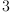(1)
Type: PositiveInteger?
fricas
B1:=OrderedVariableList [e[i] for i in 1..n]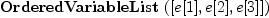(2)
Type: Type
fricas
R1:=Expression Integer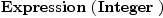(3)
Type: Type
fricas
M1:=FreeModule(R1, B1)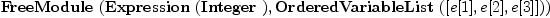(4)
Type: Type
fricas
TA1:=TensorAlgebra(M1,R1,B1)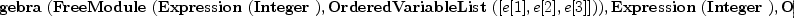(5)
Type: Type
fricas
e:=[a::TA1 for a in enumerate()$B1]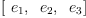(6) Type: List(TensorAlgebra?(FreeModule(Expression(Integer),OrderedVariableList([e,e,e])),Expression(Integer),OrderedVariableList([e,e,e]))) fricas T1:=x*e.1+y*e.2-z*e.3(7) Type: TensorAlgebra?(FreeModule(Expression(Integer),OrderedVariableList([e,e,e])),Expression(Integer),OrderedVariableList([e,e,e])) fricas T11:=T1*T1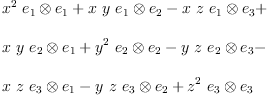(8) Type: TensorAlgebra?(FreeModule(Expression(Integer),OrderedVariableList([e,e,e])),Expression(Integer),OrderedVariableList([e,e,e])) fricas --- B2:=OrderedObjectList(BOP, map(operator,[a,b,c]))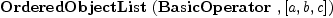(9) Type: Type fricas R2:=DeRhamComplex(Integer,[x,y,z])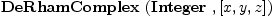(10) Type: Type fricas M2:=FreeModule(R2, B2)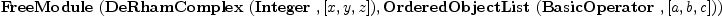(11) Type: Type fricas TA2:=TensorAlgebra(M2,R2,B2)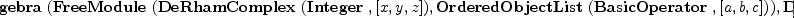(12) Type: Type fricas g:=[a::TA2 for a in enumerate()$B2]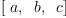(13)
Type: List(TensorAlgebra?(FreeModule(DeRhamComplex?(Integer,[x,y,z]),OrderedObjectList?(BasicOperator?,[a,b,c])),DeRhamComplex?(Integer,[x,y,z]),OrderedObjectList?(BasicOperator?,[a,b,c])))
fricas
h:=[generator(i)\$R2 for i in 1..3]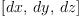(14)
Type: List(DeRhamComplex?(Integer,[x,y,z]))
fricas
T2:=h.1*g.1+h.2*g.2-h.3*g.3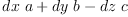(15)
Type: TensorAlgebra?(FreeModule(DeRhamComplex?(Integer,[x,y,z]),OrderedObjectList?(BasicOperator?,[a,b,c])),DeRhamComplex?(Integer,[x,y,z]),OrderedObjectList?(BasicOperator?,[a,b,c]))
fricas
T3:=g.2 * T2 - T2 * g.1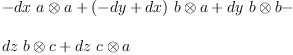(16)
Type: TensorAlgebra?(FreeModule(DeRhamComplex?(Integer,[x,y,z]),OrderedObjectList?(BasicOperator?,[a,b,c])),DeRhamComplex?(Integer,[x,y,z]),OrderedObjectList?(BasicOperator?,[a,b,c]))
fricas
T4:=h.2 * T3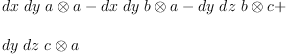(17)
Type: TensorAlgebra?(FreeModule(DeRhamComplex?(Integer,[x,y,z]),OrderedObjectList?(BasicOperator?,[a,b,c])),DeRhamComplex?(Integer,[x,y,z]),OrderedObjectList?(BasicOperator?,[a,b,c]))
fricas
)show TENSALG
TensorAlgebra(M: FreeModuleCategory(R,B),R: Ring,B: OrderedSet) is a domain constructor
Abbreviation for TensorAlgebra is TENSALG
This constructor is exposed in this frame.
------------------------------- Operations --------------------------------
?*? : (%, %) -> %                     ?*? : (Integer, %) -> %
?*? : (B, %) -> %                     ?*? : (FreeMonoid(B), R) -> %
?*? : (R, FreeMonoid(B)) -> %         ?*? : (%, R) -> %
?*? : (R, %) -> %                     ?*? : (PositiveInteger, %) -> %
?+? : (%, %) -> %                     ?-? : (%, %) -> %
-? : % -> %                           ?=? : (%, %) -> Boolean
1 : () -> %                           0 : () -> %
?^? : (%, PositiveInteger) -> %       annihilate? : (%, %) -> Boolean
antiCommutator : (%, %) -> %          associator : (%, %, %) -> %
coef : (%, FreeMonoid(B)) -> R        coef : (%, %) -> R
coefficients : % -> List(R)           coerce : Integer -> %
coerce : R -> %                       coerce : FreeMonoid(B) -> %
coerce : B -> %                       coerce : % -> OutputForm
commutator : (%, %) -> %              constant : % -> R
constant? : % -> Boolean              hash : % -> SingleInteger
latex : % -> String                   lquo : (%, B) -> %
lquo : (%, FreeMonoid(B)) -> %        lquo : (%, %) -> %
map : ((R -> R), %) -> %              mindeg : % -> FreeMonoid(B)
mirror : % -> %                       monom : (FreeMonoid(B), R) -> %
monomial? : % -> Boolean              monomials : % -> List(%)
one? : % -> Boolean                   opposite? : (%, %) -> Boolean
quasiRegular : % -> %                 quasiRegular? : % -> Boolean
recip : % -> Union(%,"failed")        retract : % -> FreeMonoid(B)
rquo : (%, B) -> %                    rquo : (%, FreeMonoid(B)) -> %
rquo : (%, %) -> %                    sample : () -> %
varList : % -> List(B)                zero? : % -> Boolean
?~=? : (%, %) -> Boolean
?*? : (NonNegativeInteger, %) -> %
?<? : (%, %) -> Boolean if R has OAMON and FreeMonoid(B) has ORDSET or R has OAMONS and FreeMonoid(B) has ORDSET
?<=? : (%, %) -> Boolean if R has OAMON and FreeMonoid(B) has ORDSET or R has OAMONS and FreeMonoid(B) has ORDSET
?>? : (%, %) -> Boolean if R has OAMON and FreeMonoid(B) has ORDSET or R has OAMONS and FreeMonoid(B) has ORDSET
?>=? : (%, %) -> Boolean if R has OAMON and FreeMonoid(B) has ORDSET or R has OAMONS and FreeMonoid(B) has ORDSET
?^? : (%, NonNegativeInteger) -> %
characteristic : () -> NonNegativeInteger
coefficient : (%, FreeMonoid(B)) -> R
construct : List(Record(k: FreeMonoid(B),c: R)) -> %
constructOrdered : List(Record(k: FreeMonoid(B),c: R)) -> % if FreeMonoid(B) has COMPAR
convert : FreeMonoid(B) -> OutputForm
hashUpdate! : (HashState, %) -> HashState
leadingCoefficient : % -> R if FreeMonoid(B) has COMPAR
leadingMonomial : % -> % if FreeMonoid(B) has COMPAR
leadingSupport : % -> FreeMonoid(B) if FreeMonoid(B) has COMPAR
leadingTerm : % -> Record(k: FreeMonoid(B),c: R) if FreeMonoid(B) has COMPAR
leftPower : (%, PositiveInteger) -> %
leftPower : (%, NonNegativeInteger) -> %
leftRecip : % -> Union(%,"failed")
linearExtend : ((FreeMonoid(B) -> R), %) -> R if R has COMRING
listOfTerms : % -> List(Record(k: FreeMonoid(B),c: R))
max : (%, %) -> % if R has OAMON and FreeMonoid(B) has ORDSET or R has OAMONS and FreeMonoid(B) has ORDSET
min : (%, %) -> % if R has OAMON and FreeMonoid(B) has ORDSET or R has OAMONS and FreeMonoid(B) has ORDSET
mindegTerm : % -> Record(k: FreeMonoid(B),c: R)
monomial : (R, FreeMonoid(B)) -> %
numberOfMonomials : % -> NonNegativeInteger
reductum : % -> % if FreeMonoid(B) has COMPAR
retractIfCan : % -> Union(FreeMonoid(B),"failed")
rightPower : (%, PositiveInteger) -> %
rightPower : (%, NonNegativeInteger) -> %
rightRecip : % -> Union(%,"failed")
sh : (%, %) -> % if R has COMRING
sh : (%, NonNegativeInteger) -> % if R has COMRING
smaller? : (%, %) -> Boolean if R has COMPAR and FreeMonoid(B) has COMPAR or R has OAMON and FreeMonoid(B) has ORDSET or R has OAMONS and FreeMonoid(B) has ORDSET
subtractIfCan : (%, %) -> Union(%,"failed")
sup : (%, %) -> % if R has OAMONS and FreeMonoid(B) has ORDSET
support : % -> List(FreeMonoid(B))

 Subject:   Be Bold !! ( 14 subscribers )